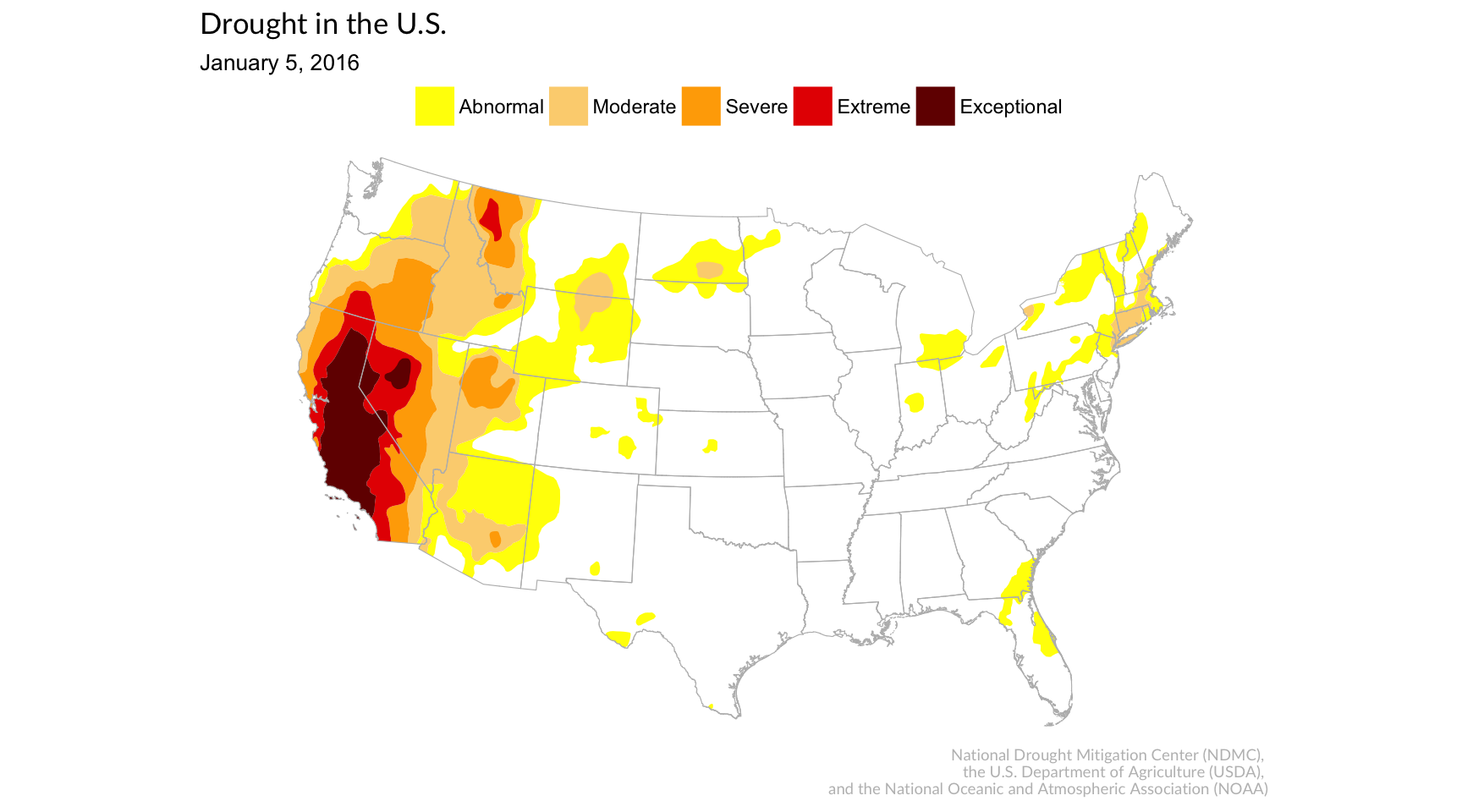This repository contains source shapefiles and visualization scripts for the Trend CT story: How the drought has spread in Connecticut .

What’s in this walkthrough:

• Generating individual visualizations of the severity of droughts in 2016
• Generating GIFs using the individual visualizations
• For both U.S. and CT

``````library(tidyr)
library(dplyr)
library(ggmap)
library(ggplot2)
library(rgdal)
library(sp)
library(maptools)
library(scales)
library(extrafont)
library(lubridate)
library(magick)``````

## Prepping the shape files

``````# Bring in the base layer of US state borders
``````## OGR data source with driver: ESRI Shapefile
## Source: "us_shape", layer: "statesp010g"
## with 61 features
## It has 11 fields``````
``````# Convert the shapefile into a dataframe that we can work with
usfort <- fortify(usmap, region="STATE_ABBR")

# NOTE: YOU CAN CREATE STATE-SPECIFIC MAPS FOR YOUR STATE IF YOU SUB OUT "CT"
# AND THEN CHANGE THE BOUNDING BOX LATITUDES AND LONGITUDES (XLIM AND YLIM IN COORD_MAP)
# IN THE CONNECTICUT CHUNK OF CODE BELOW

# Creating a shapefile specifically for Connecticut
ctfort <- filter(usfort, id=="CT")

# Creating a shapefile of all state shapes minus Connecticut
not_ct_fort <- filter(usfort, id!="CT")

# Compiling a list of the folders in the 'shapes' folder
shape_list <- list.files("shapes")``````

## United States

``````for (i in 1:length(shape_list)) {

# Loop through and gets the folder name
file_name <- shape_list[i]

# Figures out the exact folder location
dsn_name <- paste0("shapes/", file_name)

# The shapefile itself is the same as the directory minus "_M"
layer_name <- gsub("_M", "", file_name)

# The shapefiles/directories are labeled by date, so we want to extract that and turn it into a string (AP format)
the_date <- gsub("USDM_", "", layer_name)
the_date <- ymd(the_date)
ap_date <- paste0(month(the_date, label=T, abbr=F), " ", day(the_date), ", ", year(the_date))

# Now that we've got the name of the shapefile and the folder location, we can read in the data

# Transform the shapefile into a dataframe so we can work with it in R
droughtfort <- fortify(droughtmap, region="DM")

gg <- ggplot()

# This line will bring in the drought shapefile
gg <- gg +  geom_polygon(data = droughtfort, aes(x=long, y=lat, group=group, fill=id), color = "black", size=0)

# This line brings in the US borders as a layer
gg <- gg +  geom_polygon(data = usfort, aes(x=long, y=lat, group=group, fill=total), color = "gray73", fill=NA, size=0.2)

# This sets the boundaries of the view so it excludes Alaska and Hawaii
gg <- gg +  coord_map("polyconic", xlim=c(-125, -70), ylim=c(25.5, 48.5))

# This line sets the colors and text for the legend items
gg <- gg +  scale_fill_manual(name="", values = c("#FFFF00", "#FCD37F", "#FFAA00", "#E60000", "#730000"),
labels = c("Abnormal", "Moderate", "Severe", "Extreme", "Exceptional"))

# The rest of the code in this chunk is for blurbs and style.
gg <- gg + labs(x=NULL, y=NULL,
title="Drought in the U.S.",
subtitle=ap_date,
caption="National Drought Mitigation Center (NDMC), \nthe U.S. Department of Agriculture (USDA), \n and the National Oceanic and Atmospheric Association (NOAA)")
gg <- gg + theme(plot.title=element_text(face="bold", family="Lato Regular", size=13))
gg <- gg + theme(plot.caption=element_text(face="bold", family="Lato Regular", size=7, color="gray", margin=margin(t=10, r=80)))
gg <- gg + theme(legend.position="top")
gg <- gg + theme(axis.line =  element_blank(),
axis.text =  element_blank(),
axis.ticks =  element_blank(),
panel.grid.major = element_blank(),
panel.grid.minor = element_blank(),
panel.border = element_blank(),
panel.background = element_blank())
print(gg)
}``````
``````## OGR data source with driver: ESRI Shapefile
## Source: "shapes/USDM_20160105_M", layer: "USDM_20160105"
## with 5 features
## It has 4 fields````````````## OGR data source with driver: ESRI Shapefile
## Source: "shapes/USDM_20160112_M", layer: "USDM_20160112"
## with 5 features
## It has 4 fields``````